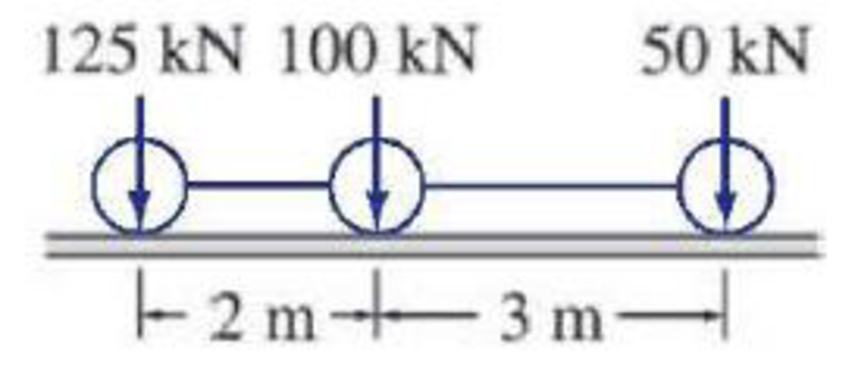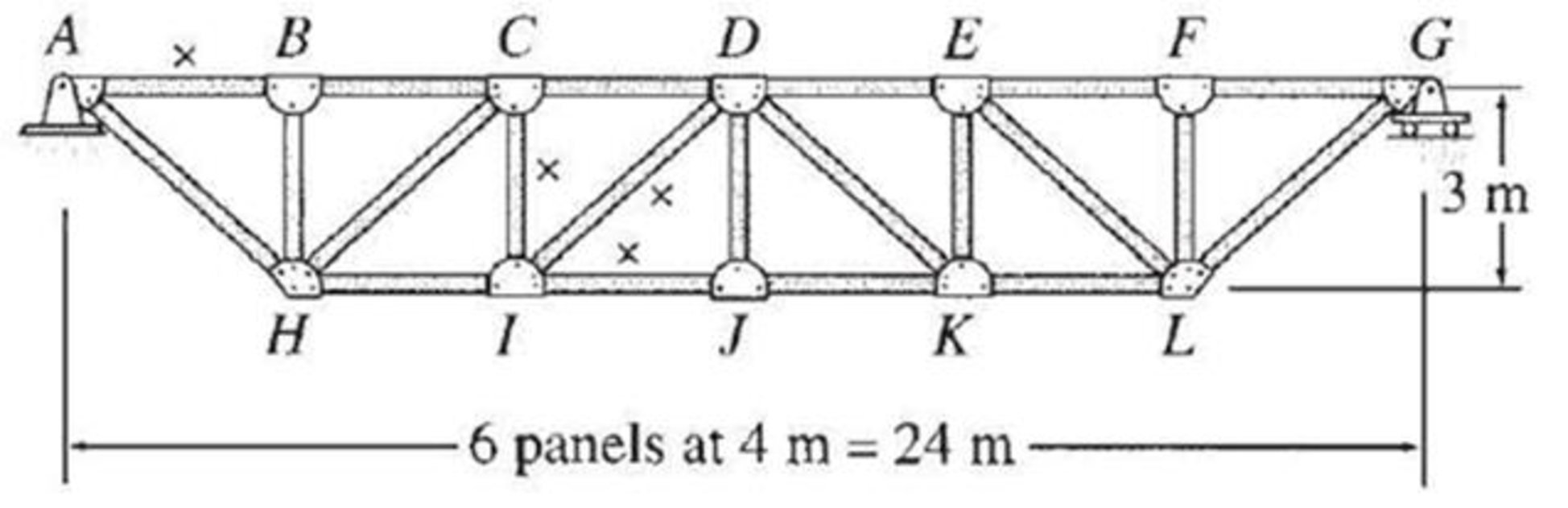# For the truss of Problem 8.53, determine the maximum tensile axial force in member DI due to the series of three moving concentrated loads shown in Fig. P9.13. FIG. P9.13, P9.17, P9.18, P9.22 FIG. P8.53

#### Solutions

Chapter
Section
Chapter 9, Problem 17P
Textbook Problem
15 views

## For the truss of Problem 8.53, determine the maximum tensile axial force in member DI due to the series of three moving concentrated loads shown in Fig. P9.13.FIG. P9.13, P9.17, P9.18, P9.22FIG. P8.53

To determine

Find the maximum tensile axial force in member DI.

### Explanation of Solution

Calculation:

Find the support reactions.

Apply 1 kN moving load from A to G in the top chord member.

Draw the free body diagram of the member as in Figure 1.

Refer Figure 1.

Find the reaction at A and G when 1 kN load placed from A to G. (0x24m).

Apply moment equilibrium at A.

ΣMA=01(x)+Gy(24)=024Gy=xGy=x24

Apply force equilibrium equation along vertical.

Consider the upward force as positive (+) and downward force as negative ().

ΣFy=0Ay+Gy1=0Ay+x241=1Ay=1x24

Influence line for the force in member DI.

The expressions for the member force FDI can be determined by passing an imaginary section aa through the members CD, DI, and IJ and then apply a vertical force equilibrium.

Draw the free body diagram as shown in Figure 2.

Refer Figure 2.

Find the equation of member force DI.

Apply a 1 kN load at just left of C (0x8m).

Consider the section DG.

Apply force equilibrium equation along vertical.

Consider upward force as positive and downward force as negative.

ΣFy=0GyFDI(35)=035FDI=GyFDI=53Gy

Substitute x24 for Gy.

FDI=53(x24)=5x72

Apply a 1 kN load at just right of C (8mx24m).

Consider the section AC.

Apply force equilibrium equation along vertical.

Consider upward force as positive and downward force as negative.

ΣFy=0Ay+FDI(35)=035FDI=AyFDI=53Ay

Substitute 1x24 for Ay.

FDI=53(1x24)=53+5x72

Thus, the equation of force in the member DI,

FDI=5x72, 0x8m        (1)

FDI=53+5x72, 8mx24m        (2)

Find the force in member DI using the Equation (1) and (2) and then summarize the value in Table 1.

 x (m) Apply 1 kN load Force in member DI (FDI) Influence line ordinate for the force in member DI (kN/kN) 0 A 0 0 8 C 5(8)72=0.556 0.556 12 D −53+5(12)72=−0.833 −0.833 24 G −53+5(24)72=0 0

Sketch the influence line diagram for ordinate for the force in member DI using Table 1 as shown in Figure 3.

Refer Figure 3.

Find the slope (θAC) of influence line diagram for the force in member DI.

θAC=0.556LAC

Here, LAC is the length of the truss from A to C.

Substitute 8 m for LAC.

θAC=0.5568=114.4

Find the slope (θCD) of influence line diagram for the force in member DI.

θCD=0.556+0.833LCD

Here, LCD is the length of the truss from C to D.

Substitute 4 m for LCD.

θCD=0.556+0.8334=12.88

The slope of portion C to O is positive and O to D is negative.

Find the distance of OD using similar triangle rule.

LOD0.833=2.881LOD=2.4m

Find the distance of CO.

LCO=LCDLOD

Substitute 4 m for LCD and 2

### Still sussing out bartleby?

Check out a sample textbook solution.

See a sample solution

#### The Solution to Your Study Problems

Bartleby provides explanations to thousands of textbook problems written by our experts, many with advanced degrees!

Get Started

Find more solutions based on key concepts
Explain why the mean of a data set is not a good way of defining the dispersion of the reported data.

Engineering Fundamentals: An Introduction to Engineering (MindTap Course List)

What are the advantages of open source software?

Enhanced Discovering Computers 2017 (Shelly Cashman Series) (MindTap Course List)

Suggest three typical business situations where referential integrity avoids data problems.

Systems Analysis and Design (Shelly Cashman Series) (MindTap Course List)

What is the Turing Test?

Fundamentals of Information Systems

Why is it important to have a planned maintenance program for tools and equipment?

Welding: Principles and Applications (MindTap Course List)

If your motherboard supports ECC DDR3 memory, can you substitute non-ECC DDR3 memory?

A+ Guide to Hardware (Standalone Book) (MindTap Course List)